# Branch And Bound | Set 4 (Job Assignment Problem)

Branch And Bound (Job Assignment Problem) - Branch And Bound - It is required to perform all jobs by assigning exactly one worker to each job.

Let there be N workers and N jobs. Any worker can be assigned to perform any job, incurring some cost that may vary depending on the work-job assignment. It is required to perform all jobs by assigning exactly one worker to each job and exactly one job to each agent in such a way that the total cost of the assignment is minimized.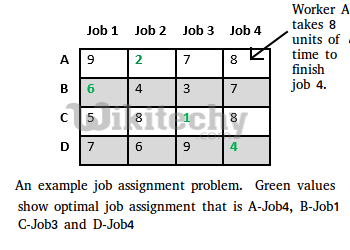Let us explore all approaches for this problem.

Solution 1: Brute Force
We generate n! possible job assignments and for each such assignment, we compute its total cost and return the less expensive assignment. Since the solution is a permutation of the n jobs, its complexity is O(n!).

Solution 2: Hungarian Algorithm
The optimal assignment can be found using the Hungarian algorithm. The Hungarian algorithm has worst case run-time complexity of O(n^3).

Solution 3: DFS/BFS on state space tree
A state space tree is a N-ary tree with property that any path from root to leaf node holds one of many solutions to given problem. We can perform depth-first search on state space tree and but successive moves can take us away from the goal rather than bringing closer. The search of state space tree follows leftmost path from the root regardless of initial state. An answer node may never be found in this approach. We can also perform a Breadth-first search on state space tree. But no matter what the initial state is, the algorithm attempts the same sequence of moves like DFS.

Solution 4: Finding Optimal Solution using Branch and Bound
The selection rule for the next node in BFS and DFS is “blind”. i.e. the selection rule does not give any preference to a node that has a very good chance of getting the search to an answer node quickly. The search for an optimal solution can often be speeded by using an “intelligent” ranking function, also called an approximate cost function to avoid searching in sub-trees that do not contain an optimal solution. It is similar to BFS-like search but with one major optimization. Instead of following FIFO order, we choose a live node with least cost. We may not get optimal solution by following node with least promising cost, but it will provide very good chance of getting the search to an answer node quickly.

READ  Java Programming - Cutting a Rod

There are two approaches to calculate the cost function:

1. For each worker, we choose job with minimum cost from list of unassigned jobs (take minimum entry from each row).
2. For each job, we choose a worker with lowest cost for that job from list of unassigned workers (take minimum entry from each column).

In this article, the first approach is followed.

Let’s take below example and try to calculate promising cost when Job 2 is assigned to worker A.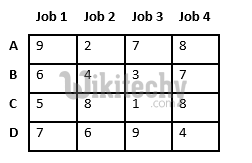Since Job 2 is assigned to worker A (marked in green), cost becomes 2 and Job 2 and worker A becomes unavailable (marked in red).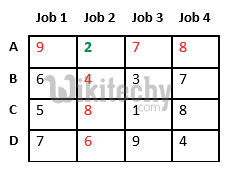Now we assign job 3 to worker B as it has minimum cost from list of unassigned jobs. Cost becomes 2 + 3 = 5 and Job 3 and worker B also becomes unavailable.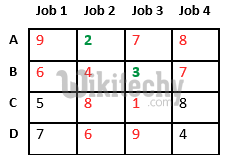Finally, job 1 gets assigned to worker C as it has minimum cost among unassigned jobs and job 4 gets assigned to worker C as it is only Job left. Total cost becomes 2 + 3 + 5 + 4 = 14.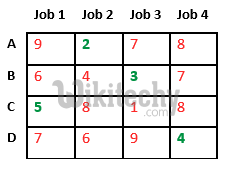Below diagram shows complete search space diagram showing optimal solution path in green.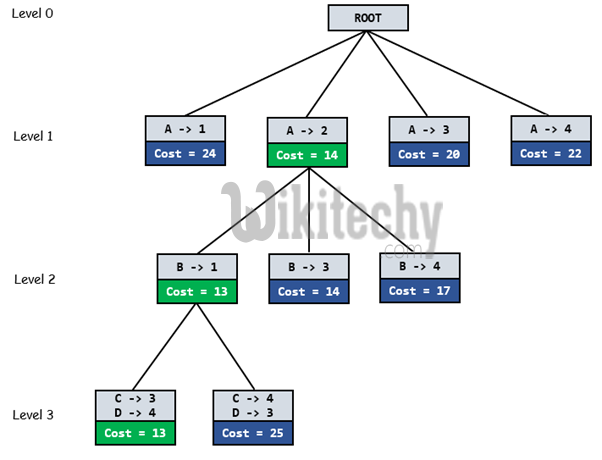Complete Algorithm:

```/* findMinCost uses Least() and Add() to maintain the
list of live nodes

Least() finds a live node with least cost, deletes
it from the list and returns it

Add(x) calculates cost of x and adds it to the list
of live nodes

Implements list of live nodes as a min heap */

// Search Space Tree Node
node
{
int job_number;
int worker_number;
node parent;
int cost;
}

// Input: Cost Matrix of Job Assignment problem
// Output: Optimal cost and Assignment of Jobs
algorithm findMinCost (costMatrix mat[][])
{
// Initialize list of live nodes(min-Heap)
// with root of search tree i.e. a Dummy node
while (true)
{
// Find a live node with least estimated cost
E = Least();

// The found node is deleted from the list
// of live nodes
if (E is a leaf node)
{
printSolution();
return;
}

for each child x of E
{
Add(x); // Add x to list of live nodes;
x->parent = E; // Pointer for path to root
}
}
}```

Below is its C++ implementation.

``````// Program to solve Job Assignment problem
// using Branch and Bound
#include <bits/stdc++.h>
using namespace std;
#define N 4

// state space tree node
struct Node
{
// stores parent node of current node
// helps in tracing path when answer is found
Node* parent;

// contains cost for ancestors nodes
// including current node
int pathCost;

// contains least promising cost
int cost;

// contain worker number
int workerID;

// contains Job ID
int jobID;

// Boolean array assigned will contains
// info about available jobs
bool assigned[N];
};

// Function to allocate a new search tree node
// Here Person x is assigned to job y
Node* newNode(int x, int y, bool assigned[],
Node* parent)
{
Node* node = new Node;

for (int j = 0; j < N; j++)
node->assigned[j] = assigned[j];
node->assigned[y] = true;

node->parent = parent;
node->workerID = x;
node->jobID = y;

return node;
}

// Function to calculate the least promising cost
// of node after worker x is assigned to job y.
int calculateCost(int costMatrix[N][N], int x,
int y, bool assigned[])
{
int cost = 0;

// to store unavailable jobs
bool available[N] = {true};

// start from next worker
for (int i = x + 1; i < N; i++)
{
int min = INT_MAX, minIndex = -1;

// do for each job
for (int j = 0; j < N; j++)
{
// if job is unassigned
if (!assigned[j] && available[j] &&
costMatrix[i][j] < min)
{
// store job number
minIndex = j;

// store cost
min = costMatrix[i][j];
}
}

// add cost of next worker
cost += min;

// job becomes unavailable
available[minIndex] = false;
}

return cost;
}

// Comparison object to be used to order the heap
struct comp
{
bool operator()(const Node* lhs,
const Node* rhs) const
{
return lhs->cost > rhs->cost;
}
};

// print Assignments
void printAssignments(Node *min)
{
if(min->parent==NULL)
return;

printAssignments(min->parent);
cout << "Assign Worker " << char(min->workerID + 'A')
<< " to Job " << min->jobID << endl;

}

// Finds minimum cost using Branch and Bound.
int findMinCost(int costMatrix[N][N])
{
// Create a priority queue to store live nodes of
// search tree;
priority_queue<Node*, std::vector<Node*>, comp> pq;

// initailize heap to dummy node with cost 0
bool assigned[N] = {false};
Node* root = newNode(-1, -1, assigned, NULL);
root->pathCost = root->cost = 0;
root->workerID = -1;

// Add dummy node to list of live nodes;
pq.push(root);

// Finds a live node with least cost,
// add its childrens to list of live nodes and
// finally deletes it from the list.
while (!pq.empty())
{
// Find a live node with least estimated cost
Node* min = pq.top();

// The found node is deleted from the list of
// live nodes
pq.pop();

// i stores next worker
int i = min->workerID + 1;

// if all workers are assigned a job
if (i == N)
{
printAssignments(min);
return min->cost;
}

// do for each job
for (int j = 0; j < N; j++)
{
// If unassigned
if (!min->assigned[j])
{
// create a new tree node
Node* child = newNode(i, j, min->assigned, min);

// cost for ancestors nodes including current node
child->pathCost = min->pathCost + costMatrix[i][j];

// calculate its lower bound
child->cost = child->pathCost +
calculateCost(costMatrix, i, j, child->assigned);

// Add child to list of live nodes;
pq.push(child);
}
}
}
}

// Driver code
int main()
{
// x-cordinate represents a Worker
// y-cordinate represents a Job
int costMatrix[N][N] =
{
{9, 2, 7, 8},
{6, 4, 3, 7},
{5, 8, 1, 8},
{7, 6, 9, 4}
};

/* int costMatrix[N][N] =
{
{82, 83, 69, 92},
{77, 37, 49, 92},
{11, 69,  5, 86},
{ 8,  9, 98, 23}
};
*/

/* int costMatrix[N][N] =
{
{2500, 4000, 3500},
{4000, 6000, 3500},
{2000, 4000, 2500}
};*/

/*int costMatrix[N][N] =
{
{90, 75, 75, 80},
{30, 85, 55, 65},
{125, 95, 90, 105},
{45, 110, 95, 115}
};*/

cout << "\nOptimal Cost is "
<< findMinCost(costMatrix);

return 0;
}
Run on IDE``````

Output :

```Assign Worker A to Job 1
Assign Worker B to Job 0
Assign Worker C to Job 2
Assign Worker D to Job 3

Optimal Cost is 13```

#### About the author#### Venkatesan Prabu

Wikitechy Founder, Author, International Speaker, and Job Consultant. My role as the CEO of Wikitechy, I help businesses build their next generation digital platforms and help with their product innovation and growth strategy. I'm a frequent speaker at tech conferences and events.

X